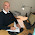## måndag 8 mars 2021

### Why Using Complex Numbers for Real Physics?

The mathematical modelling of wave phenomena, in particular in electromagnetics, usually uses complex numbers with time dependence captured in exponential factors with $i$ the imaginary unit

• $\exp(i\omega t) = \cos(\omega t) + i*\sin(\omega t)$
with $t$ time and $\omega$ frequency and $*$ multiplication. This is useful because multiplication of exponential factors is direct, but in the end the computation is brought back to physical real-valuedness by collecting real and imaginary parts. In principle the mathematical model thus can take as well real-valued form and the use of complex numbers is a technicality but not something of fundamental importance for physics.

The Schrödinger equation in atom physics is usually expressed in complex (normalised) form as
• $i*\frac{\partial\Psi}{\partial t} + H\psi =0$,       (1)
where $\Psi (x,t)$ is a complex-valued wave function depending on one (or several space) variables $x$ and $H$ is a Hermitian (self-adjoint/symmetric)  "real-valued" operator acting on wave functions. Splitting $\Psi$ into real and imaginary part $\Psi = \psi + i*\phi$ with $\psi$ and $\phi$ real-valued, we can write (1) as the following system in real-valued functions:
• $\frac{\partial\psi}{\partial t} + H\phi =0$         (2a)
• $\frac{\partial\phi}{\partial t} - H\psi =0$          (2b)
which carries the same physics as (1).

There is a lot of mystery with the Schrödinger equation, part of which connects to the appearance of the mysterious imaginary unit $i$ in (1). Sabine Hossenfelder takes up a discussion asking Do Complex Numbers Exist? The upshot is a claim that certain forms of quantum mechanical "entanglement" can only appear in Schrödinger's equations expressed in complex form. Whether this is real physics is not made clear.

The equation (2) express mechanics of waves consisting of oscillations between $\phi$ and $\psi$ much like the following reduced version of (2)
• $\frac{\partial\psi}{\partial t} + \omega\phi =0$         (3a)
• $\frac{\partial\phi}{\partial t} - \omega\psi =0$        (3b)

#### modelling a harmonic oscillator with solution $\phi(t) = \sin(\omega t)$ and $\psi(t) = \cos(\omega t)$.

The corresponding solution of (2) takes the form $\phi(x,t) = \sin(\omega t)\bar\phi (x)$ and $\psi(x,t) = \cos(\omega t)\bar\psi (x)$ where $\bar\phi =\bar\psi$ is an eigenfunction of $H$ satisfying $H\bar\phi =\omega\bar\phi$.

#### Electrons cannot swirl around an atomic kernel, because then they would radiate and the atom would not persist. On the other hand, electrons cannot be fully stationary, since then an atom would not be able to radiate subject to forcing.

The key is to understand that in an atom in ground state (or higher eigenmodes) the electron density is stationary, which means that the atom is in a state without radiation an as such can exist over time, while superposition of different eigenmodes involves variation of electron density allowing interaction with the environment in terms of radiation.  This is commonly described as "electrons jumping between different levels" which is not a very happy physical description.

#### 2 kommentarer:

1.Check out my article for Grey Market Premium of Laxmi Organic IPO GMP

1.What does your article have to do with what Professor Johnson writes?